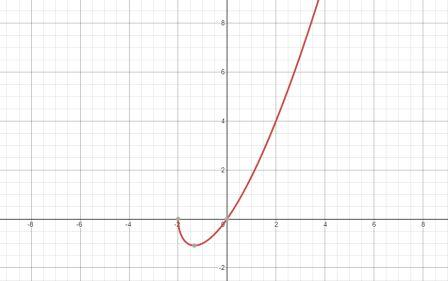# Sketch the function f(x) = x \sqrt{2+x} Investigate the following graphical information a....

## Question:

Sketch the function {eq}f(x) = x \sqrt{2+x} {/eq}

Investigate the following graphical information

a. Domain

b. Intercepts

c. Symmetry

d. Asymptotes

e. Concavity and points of inflection

## Analyzing the Graph of a Function

This problem involves the analysis of the given function. We will use the definitions of elements like domain, range, intercepts etc to extract the desired information. To find the points of inflection we are going to make use of the second derivative.

We have,

{eq}f(x) = x \sqrt{2+x} {/eq}

The graph below shows our function:a. Domain

Domain is the set of the values of those real numbers...

Become a Study.com member to unlock this answer! Create your account